HomeBlog

# Analysis of Calculation Theory for Transformer Temperature Rise

Summary

Based on heat balance principle, the basic theory for calculation of steady and transient temperature rise of transformer is discussed.

The calculation of transformer temperature rise is mainly used to make sure that the steady-state temperature rises produced by the transformer with rated load in long-term continuous operation will not exceed the limit specified in the standard or technical contract. In addition, the data of overload operation capacity of transformer running under various rated loads are also used to offer support to the measures taken to ensure safe overload operation of electrical systems.

However, from the author's current reading of recent info on transformer principles, design and calculation theories available, it may be due to the limitation of space or different aspects of emphasis that they are always making introduction to various practical formulas for transformer temperature rise calculation in a practical way. As to the involved thermal principle of calculating the transformer temperature rise is always a bit of an oversimplification i think, especially the thermal analyses demonstrating how heat energy be absorbed(or dissipate) and temperature goes up(or goes down) during a heating process. In this article, therefore i would like to make some theoretical discussions to solve this problem, and add some extra explanations needed.

 Core Transformer temperature rise calculation Purpose Ensure safe overload operation English name Transformer Category Static electrical device Function Increasing or decreasing the alternating voltages Feature 100% efficient

Catalogs

 Catalogs 1. Foreword 2.3 Just as same as the situation of 2.1, but Τ≠0 when t=0 3.3 Definition, function and derivation of the thermal time constant Τ 2. Heating & cooling process 3. Analyses of some formulas mentioned in Section 2 4.Concluding Remarks 2.1 Heating process when the thermal power is constant 3.1 Mechanism of temperature-rising process of heated body 2.2 The cooling process in which the heated body is no longer supplied with heat when the temperature rise reaches the value of Τu 3.2 Properties and applications of Τu in formula 2

Introduction

1. Foreword

It is well known that heat is always transferred automatically from a high-temperature object to a low-temperature object. Heat can transfer (or move) in three ways: conduction, convection and radiation, usually a combination two or three of them may cause the transmission (also known as heat dissipation).

Heat Transfer: Conduction, Convection, Radiation

Before formally describing this section, I would like to quote two conclusions from laboratory research that are fundamental to thermal science:

1) the temperature rise of an object is directly proportional to the heat (heat) supplied by the outside world, and inversely proportional to its own mass and Specific Heat Capacity.

2) the heat energy (calorific energy) that a heating object disperses into a cooler object(cooling medium) within a unit time is directly proportional to the area of its radiating surface, the temperature difference and the Heat Transfer Coefficient between the heating object and cooling medium.

Specific heat capacity: the ratio of the heat added to (or removed from) an object to the resulting temperature change per unit mass of a material.

Heat transfer coefficient: when the temperature difference between the heating object and the cooling object is 1K, the heat from the unit dissipation surface area to the cooler object within a unit time.

For the convenience of discussion, the heating object is referred to as the heated body in this paper, and the object which gets the heat released from the heated body is called the cooling body (or the cooling medium).

Take dry transformer for example,its winding and core are heating bodies, and cooling body refers to the air around the transformer. For oil-immersed transformers, in addition to the winding and core known as heating bodies, the oil filled in the transformer can also be called a heating one in relation to ambient air or cooling water. However, relative to the winding and core, oil is also called a cooling body(cooling medium).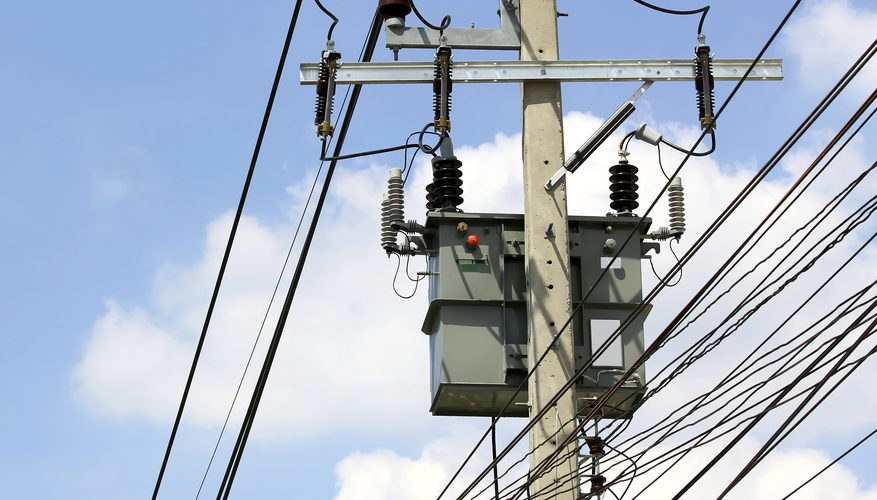Fig. 1 Transformers are found everywhere alternating current is used

Detail

2. Heating & cooling process

2.1 Heating process when the thermal power is constant

Suppose that in the heating process (that is, the heat is still in a transition state, not reaches stable state yet), the temperature rise by Τ relative to the cooling medium, the temperature rise increases dΤafter a tiny time unit dt.

The heat supplied by the outside during this dt period is a constant thermal power of Pdt(P. For windings in oil-immersed transformers, it refers to load loss; for oil, it refers to total loss). Let the heat supplied by the outside surroundings during this dt period be Pdt(P is the constant thermal power; for windings in oil-immersed transformer, P refers to load losses and for oil, the total losses). Some of the Pdt is the heat absorbed when the temperature rise of the heated body increases by dΤ, and the other part is dispersed into the cooling medium.

In order to understand the nature of this physical phenomena in the heating process better, suppose heated body is an isothermic one, therefore the density, specific heat capacity and any other parameters being the same, including the heat dissipation capacity of each point on the surface.

According to the principle of thermal balance (it comes down to the law of energy conservation), the states of the heating process described above can be represented by the following equations: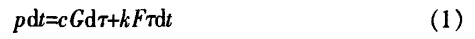Where

P——Constant value of thermal power

c——Specific heat capacity of heated body

G——mass of heated body

F——Heat dissipation surface area of heated body

k——Heat transfer coefficient

Τ——Temperature difference (or temperature rise) between the heated body and cooling body at a certain time

The first item on the right of Equation (1) indicates the heat energy absorbed by the heated body when it increases the temperature of dΤ; The second item indicates that the heat energy is dispersing to the cooling body while the heated body is storing heat.

When the temperature rise of the heat body does not go up, that is, when the temperature rise reaches a stable state, then there is dΤ=0 and Τ=Τuu means the steady-state temperature rise).

At this point, from formula (1) there is:According to the above analyses we can see: formula (1) is the mathematical expression of the heat balance principle under transient state; formula (2) is the mathematical expression of the heat balance principle under steady state.

From Formula (1) and Formula(2) we can get a first order differential equation of Τ: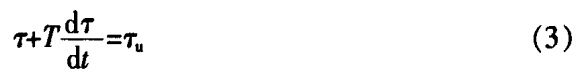Where: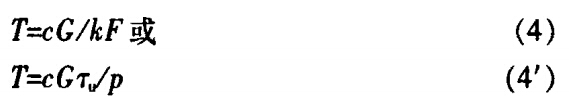From formula (4), the parameters on the right side of the equation are the physical parameters of the object itself, so Τ is a constant and has a dimension of time, so it is called the thermal time constant. In the physical sense, Τ is the ratio of the heat storage capacity of heated body to the heat dissipation capacity per unit time of the heating system studied, which is an attribute of heated body. Therefore formula (4) is considered to be the definition of Τ and the formula (4') is another expression for calculation.

To obtain the solution of formula (3) we let t=0 and Τ=0, then this is what we get: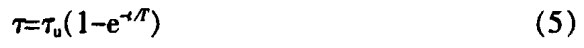Note that sometimes it is easier to express the formula (5) with temperature θ instead of temperature rise Τ, so it is reworded as follows: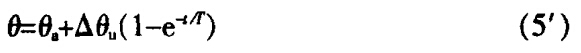Where:

θ——The temperature of heated body at any given moment and there is θ-θа

θа——The temperature of cooling body

θu——The steady state temperature of the heated body that reaches a steady state

Δθu——θuаu

The rising curve in Fig. 1 shows the temperature rise of heated body changes with time t.

In order to visually see how the Τ changes with the temperature rise, Fig. 1  shows two curves with different Τ and same Τu.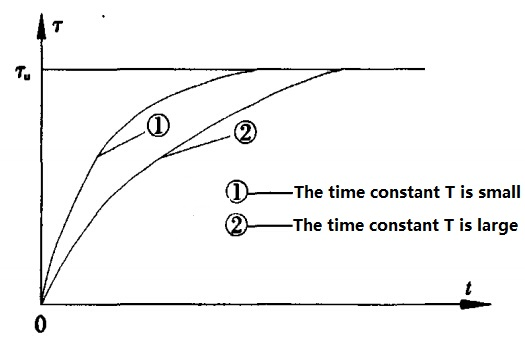Fig. 2 Relation between temperature-rise(Τ)of heated body and time(t)

As can be seen directly from formula (5), formula (5') and Fig. 1, the process of temperature rising of heated body is characterized by the fact that it changes fast at the very start, then gradually slows down, and when the time t becomes equals to (4～6)Τ, it remains almost unchanged, at this point it can be assumed that Τ reaches Τ(theoretically t reaches ∞).

2.2 The cooling process in which the heated body is no longer supplied with heat when the temperature rise reaches the value of Τu

When temperature rise of the heated reaches Τu and no heat will be emitted, the temperature rise begins to go down from Τu to zero, which we call the cooling process. At this point, its transient process equation can still be deduced by formula (1), in which you need only to let P be equal to zero.

Therefore the first order differential equation of its temperature rise is as follows: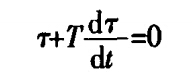The solution is: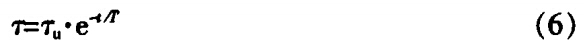All the symbols of parameter in the formula above are identical with those in formula (5), except that Τu is the initial value of temperature rise when t=0; when (4～6)Τ later, Τ≈0.

Why would we worry about Formula 6?

This is because the temperature rise of winding got at the end of current transformer temperature rise test, is still the value of temperature rise calculated through measurements of resistance value of the winding which will change with the temperature. The resistance value measurement is still carried out in the temperature rise test under a way of supply voltage been removed, thus the formula (6) should be used to calculate.

2.3 Just as same as the situation of 2.1, but Τ≠0 when t=0

When the heating body is supplied with constant heat power and Τ=Τ0 (≠0) when t=0 satisfied, the whole process of deduction of temperature rise calculation, with the exception of the situation of Τ=Τ0 when t = 0, is the same as 2.1, in other words, the formula of temperature rise can still be deduced from formula (5) as follows: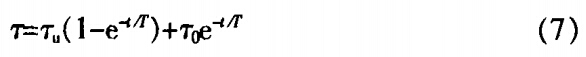Comparing this with the formula (5) we notice that there is a new second item occurs. Considering the physical meaning of the expression is not obvious enough, it is now rewritten (which will not change the result of the calculation) as: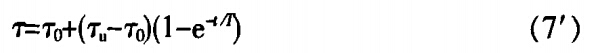These two formulas above show a case that the transformer is suddenly asked to conduct a overload operation running beyond the steady load.

Analyses

3. Analyses of some formulas mentioned in Section 2

3.1 Mechanism of temperature-rising process of heated body

Formula (5) and Fig. 1 describe the rising process of heated body temperature from a mathematical point of view. This process is characterized by a rapid start and then a gradual slow down until it finally stops rising and reaches a stable temperature rise of Τu .

In this section, characteristics of heating and cooling mechanism in this process will be described from a physical point of view. So we divide the t into n small and equal time periods when t=Τu, that is Δt1=Δt2=……=Δtn=Δt (theoretically t=∞, but in practice, it is desirable to suppose that t=(4～6)T, considering the value required of Tu with a higher accuracy). Therefore, the derivative symbol "d" in this article is replaced with a increment sign "Δ".

Please note that the heat energy supplied by surroundings in each time period is equal to P·Δt (P is the constant thermal power).

Now let us take a look in the first time period Δt1. Since the temperature of heated body has already made be equal to the cooling body when t=0, that is Τ=0, according to the second item of formula (1), we can assume the heat energy emitted is also equal to 0 during the period of Δt1 until the end of present stage. Therefore, during the Δt1 period, the final increments of the temperature rise of the heated bodyΔΤ1 is determined by the total external heat energy (P·Δt). So the temperature rise at the end of first time period Δt1 is Τ1=ΔΤ.

According to the same analytical principle, we continue with the second time period Δt2. Since the initial temperature rise at the beginning of Δt2 is the that of the first time period Δt1, it can be included that Τ1=ΔΤ1. The heat emitted during Δt2 is no longer zero, but. At the end of the Δt2, the increment value of temperature rise of heated body ΔΤ2 is determined by, so there we have ΔΤ2<ΔΤ1 (That is, the increment of temperature rise in the second period is smaller than that in the first period).

Finally, at the end of the second time period Δt2, the temperature rise Τ2 is equal to ΔΤ1+ΔΤ2.

The rest may be deduced by analogy, at the end of Δtn time period, the temperature rise is, also ΔΤn=0 has been illustrated at the same time.

According to the changes of temperature increment ΔΤn in each of time period above, there always are: ΔΤ1>ΔΤ2…>ΔΤn-1>ΔΤ(ΔΤn=0), therefore demonstrating the trend that all the heat absorbed by heated body in each time period gradually goes down from P·Δt absorbed in the time period of Δt1 to 0 absorbed in the time period of Δtn. The heat energy emitted in each of the corresponding time periods is gradually increased from 0 to(from the first time period Δt1 to the end of the NO.n time period Δtn). That also means the final temperature rise (steady temperature rise) is: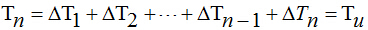After the temperature rise reaches the stable value of Τu, the heated body no longer absorbs external heat energy, which indicates that all the external heat energy has been dispersed to the cooling body.

3.2 Properties and applications of Τu in formula 2

Formula 2 is a theoretical formula derived from the heat balance principle for calculating the steady-state temperature rise of the heated body. It is difficult to calculate the temperature rise directly for complex heated bodies such as transformers. Therefore, various manufacturers and scientific research institutions have respectively obtained many practical formulas, according to their own practical experience or scientific research results and the characteristics of the heated body structure and three different heat dissipation forms, including conduction, convection and radiation. Furthermore, a heated body such as a transformers having a complex structure does not always have the characteristics of the homogeneous isothermal body assumed in the formula 2, and the heat-dissipation capability at each point on the surface is not equal, either. Therefore, the steady-state temperature rise (Τu) calculated from the formula 2 (including the relevant practical formula based on formula 2) on the whole indicates the average value of temperature rise at different points in the heat source. The calculation of the maximum temperature rise (temperature) of an object at a "hot spot" of concern has so far could only be mainly estimated by experience and the use of temperature rise measurements in certain heat tests (for example, confirming the difference between the maximum temperature rise and the average value or confirming the multiple value between them to estimate).

3.3 Definition, function and derivation of the thermal time constant Τ

Formula 4 is the definition expression of thermal time constant. It is obtained in the derivation of formula 5 from formula 2 and formula 1. Therefore, it is possible to think that equation 4 is obtained under the admission that when t=∞ it has dΤ=0 and Τ=Τu, the latter of which is obtained under the boundary conditions of objective reality. Because the definition of "Τ" and some functions have been described in section 2.1, here i only do some additional analyses to formula 7 which shows applications of transformer temperature rise under a short-time overload operation.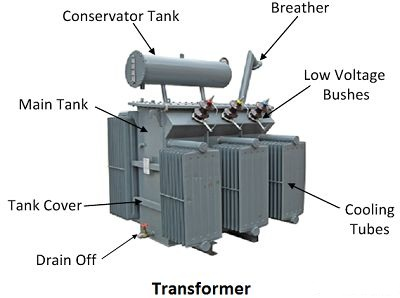Fig. 3 What is a Transformer

The basic theory of transformer and working principle of transformer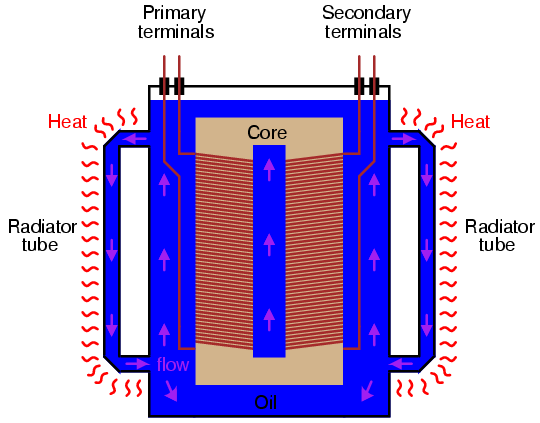Fig. 4 Large power transformers have their core and windings submerged in an oil bath to transfer heat and muffle noise, and also to displace moisture which would otherwise compromise the integrity of the winding insulation. Heat-dissipating "radiator" tubes on the outside of the transformer case provide a convective oil flow path to transfer heat from the transformer's core to ambient air.

First of all, according to the statistics of a large number of dry and oil-immersed power transformers with different capacities, which have been manufactured at home and abroad, the value of Τ is generally not less than 1h (for oil-immersed transformer, although the T of winding is quite low, about 5 min-20 mins, but the T of oil is 1h～5h. Noting that the oil actual temperature rise is generally not lower than the temperature difference between the winding and the oil, and that the thermal time constant of the oil should therefore be considered to control the temperature rise of the winding during the overload operation, that is, the temperature difference between winding and ambient air or temperature of cooling water still plays a decisive role. Although the Τu of the transformer will obviously exceed the temperature rise limit of rated-load operation, as long as the time t has been controlled within T, it can still make the actual temperature rise running in short-time overload not excess the temperature rise limit of short-time overload operation. This permissible limits, according to the standard of the load guide of the oil immersed power transformer, is related to the operation type within nameplate capacity, namely, normal periodicity, long-term or short-term emergency, and generally higher than that of rated temperature rise.

It can be seen from this that the function of the thermal time constant T is relatively large, so it is necessary to pay close attention to its definition, various affecting factors and derivation of its formula. However , i have seen some people added another boundary condition except dΤ=0 during the derivation of the expression of Τ: in extreme cases, the heat will not transferred into the surrounding medium at all, which is also called "adiabatic condition". In this regard, i would like to put forward the following different views for discussion.

(1) In this article, the derivations of formula 4 and formula 5 have only used a "boundary condition" of dΤ=0 (Τ=Τu) when t=∞, so there is no need to add another adiabatic condition for derivation.

It is said that without heat dissipation, the time required to reach a stable temperature is called time constant (Τ), but that will only be true when the second item(dissipated heat) on the right of the equation meets a condition of, now that to reach a steady temperature Τ must be equal to Τu, which is at variance with objective reality of electric accessories including transformers.

Some might say that when people calculate the temperature of a transformer at a short-circuit current, don't they also use the formula obtained under the "adiabatic condition" to calculate the temperature of the transformer? Yes, but the case being considered is only the "short time" one, that is, the formula only applies when short-circuit durations never exceed 10s (actually 2s). The time is very small compared with the thermal time constant of winding in oil-immersed power transformer (about 5min-20mins) and that of oil (about 1lh～5h). This is still true when compared with the thermal time constant of the windings of dry-type transformers (about or above 30min). In section 3.1 of this article, such a short duration makes it is possible to consider it as an adiabatic transient system. In a broad sense, if the heat energy emitted accounts for only a very small part of the heat supplied by the outside world during a same period, it can be roughly regarded as adiabatic process. At this point, it is precisely because of the recognition ofthat it is in line with objective reality.

This proves that adiabatic conditions should not be used as the basis in the derivation of expression of Τ (formula 4) and that of transient temperature rise (formula 5).

(3) From the details of the derivation process in this article, i would like to say that the true expression of temperature rise of the heated body still have not been obtained yet in the end. Think about that, a task for you, my readers.

4.Concluding Remarks

(1) Starting from the principle of heat balance, this article expounds the model of the heating mechanism in the rising process of temperature rise of heated body with concise mathematics and physical language.

(2) It is clearly pointed out in this paper that the steady temperature rise of the heated body can be calculated by using formula 2, and formula 5 and formula 7 are the formulas for calculating the temperature rise of heated body during the transient process.

(3) It is pointed out that to obtain the expression of the transient temperature rise of the thermal time constant Τ and the heated body, the adiabatic condition should not be used for the process of derivation, which is not necessary either.

(4) Due to space constraints, this article has not covered the heat dissipation mechanism of heated body, but you readers can refer to other reference about heat loss or transfer in Kynix and other sites.

Book Suggestion

Predicting Transformer Temperature Rise in the Presence of Harmonics: Improving Power Transformer Characteristics By Using K- Factor And Narrow Band Width Transformer (NBT) System Paperback – May 17, 2017

Power transformers represent the largest portion of capital investment in transmission and distribution substations. One of the most important parameters governing a transformer’s life expectancy is the hot spot temperature value. Temperature rise in a transformer depends on variety of parameters such as ambient temperature, output current and type of the core. The significance of harmonics in power systems has increased substantially due to the use of solid state controlled loads and other high frequency producing devices. An important consideration when evaluating the impact of harmonics is their effect on power system components and loads.

---by Waleed Abdel Aziz, Ossama Gouda and Ghada Amer

Power Transformers: Principles and Applications (Power Engineering (Willis))Apr 12, 2002

Complete with equations, illustrations, and tables, this book covers the basic theory of electric power transformers, its application to transformer designs, and their application in utility and industrial power systems. The author presents the principles of the two-winding transformer and its connection to polyphase systems, the origins of transformer losses, autotransformers, and three-winding transformers and compares different types of transformer coil and coil construction.

---by John Winders

Transformer Design Principles: With Applications to Core-Form Power Transformers, Second Edition 2nd Edition

With Applications to Core-Form Power Transformers, Second Edition remains focused on the basic physical concepts behind transformer design and operation. Starting with first principles, this book develops the reader’s understanding of the rationale behind design practices by illustrating how basic formulae and modeling procedures are derived and used.

---by Robert M. Del Vecchio and Bertrand Poulin

Relevant information "Analysis of Calculation Theory for Transformer Temperature Rise"

About the article "Analysis of Calculation Theory for Transformer Temperature Rise", If you have better ideas, don't hesitate to  write your thoughts in the following comment area.

You also can find more articles about electronic semiconductor through Google search engine, or refer to the following related articles:

Some suggestions about protecting transformers

Learn Some Basic Knowledge about Capacitor Voltage Transformer

### Related PostsHOW TO BUY ELECTRONIC COMPONENTS
Search
Inquiry
Order
Track

Delivery
FedEx
UPS
DHL
TNT

Payment Terms
By PayPal
By Credit Card
By Wire Transfer
By Western Union

After-sales Service
Quality Control
Guarantee
Return & Replacement